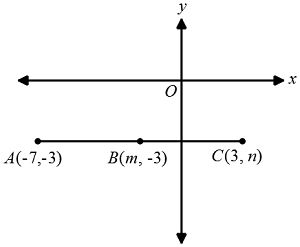# SAT Midpoint FormulaIn the figure above, point $B$ is the midpoint of segment $\overline{AC}$. What is the value of $m-n?$

(A) $\ \ -5$
(B) $\ \ -3$
(C) $\ \ -2$
(D) $\ \ 1$
(E) $\ \ 2$

×